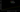# Prime Number Theorem

22 March 2009 — written by Prakash PrasadProperties of Prime Numbers have been studied as far back as the ancient Greek civilization. Be it the mathematicians of Pythagoras’ School (500 BC to 300 BC), Euclid in about 300 BC or Eratosthenes in around 200 BC; the Greeks came up with most of the basic and important identities and their proves:

• Pythagoras’ Mathematicians came up with the identity of Perfect and Amicable numbers.
• Euclid, in the Book IX of the Elements, proved that there are infinitely many prime numbers.
• Eratosthenes devised a very fast algorithm for calculating primes called the Sieve of Eratosthenes.

Then there was a very long gap in the history of prime numbers during the Dark Ages. But the work was again taken up by world famous mathematicians of the likes of Mersenne (Mersenne Primes), Euler (extended Fermat’s Little Theorem and found 60 pairs of amicable numbers), Cataldi (proved M19 in 1588), Fermat (Fermat’s Little Theorem). Legendre and Gauss both did extensive calculations on the number of primes in a certain limit, i.e. $f(n)$ is the number of primes in the interval $[1,n]$.

Gauss (who was a prodigious calculator) told a friend that whenever he had a spare 15 minutes he would spend it in counting the primes in a ‘chiliad‘ (a range of 1000 numbers). By the end of his life it is estimated that he had counted all the primes up to about 3 million.

Both Legendre and Gauss came to the conclusion that for large n the density of primes near $n$ is approximately:

$\frac{1}{\log n}$

This is known to be the Prime Number Theorem.

Many scientists came up with modifications to the formula to come closer to the actual value of $f(n)$. Legendre gave an estimate for $π(n)$ the number of primes $n$ of:

$\pi (n) = \frac{n}{\log n - 1.08366}$

while Gauss’s estimate is in terms of the logarithmic integral:

$\pi (n) = \int\limits_2^n \frac{dt}{\log t}$